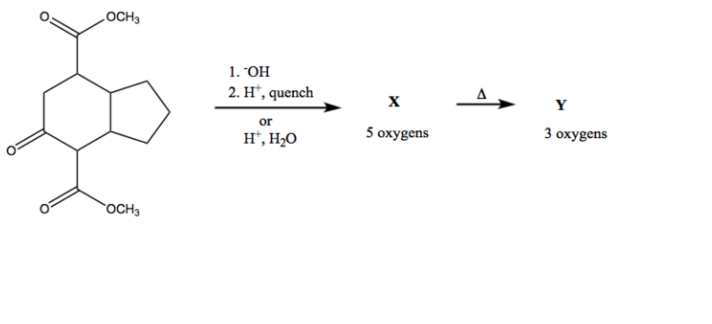# Problem: For the following scheme, draw the structures for  X and Y.

###### FREE Expert Solution
91% (171 ratings)
###### Problem Details

For the following scheme, draw the structures for  X and YFrequently Asked Questions

What scientific concept do you need to know in order to solve this problem?

Our tutors have indicated that to solve this problem you will need to apply the Carboxylic Acid Synthesis concept. If you need more Carboxylic Acid Synthesis practice, you can also practice Carboxylic Acid Synthesis practice problems.

What is the difficulty of this problem?

Our tutors rated the difficulty ofFor the following scheme, draw the structures for  X and Y. ...as low difficulty.

How long does this problem take to solve?

Our expert Organic tutor, Bart took 4 minutes and 23 seconds to solve this problem. You can follow their steps in the video explanation above.